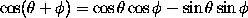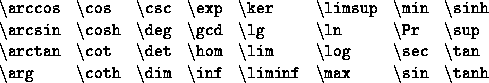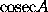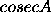### Standard Functions (sin, cos etc.)

The names of certain standard functions and abbreviations are obtained by typing a backlash \ before the name. For example, one obtainsby typing
$\cos(\theta + \phi) = \cos \theta \cos \phi - \sin \theta \sin \phi$


The following standard functions are represented by control sequences defined in LaTeX:Names of functions and other abbreviations not in this list can be obtained by converting to the roman font. Thus one obtainsby typing $\mathrm{cosec} A$. Note that if one were to type simply $cosec A$ one would obtainbecause LaTeX has treated cosec A as the product of six quantities c, o, s, e, c and A and typeset the formula accordingly.